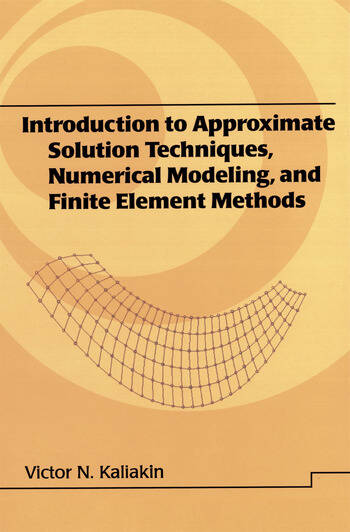# Introduction to Approximate Solution Techniques, Numerical Modeling, and Finite Element Methods

## 1st Edition

Victor N. Kaliakin

CRC Press
Published September 25, 2001
Textbook - 664 Pages
ISBN 9780824706791 - CAT# DK5652
Series: Civil and Environmental Engineering

For Instructors Request Inspection Copy

was \$205.00

USD\$164.00

SAVE ~\$41.00

FREE Standard Shipping!

## Preview

### Summary

Functions as a self-study guide for engineers and as a textbook for nonengineering students and engineering students, emphasizing generic forms of differential equations, applying approximate solution techniques to examples, and progressing to specific physical problems in modular, self-contained chapters that integrate into the text or can stand alone!

This reference/text focuses on classical approximate solution techniques such as the finite difference method, the method of weighted residuals, and variation methods, culminating in an introduction to the finite element method (FEM).

Discusses the general notion of approximate solutions and associated errors!

With 1500 equations and more than 750 references, drawings, and tables, Introduction to Approximate Solution Techniques, Numerical Modeling, and Finite Element Methods:

• Describes the approximate solution of ordinary and partial differential equations using the finite difference method
• Covers the method of weighted residuals, including specific weighting and trial functions
• Considers variational methods
• Highlights all aspects associated with the formulation of finite element equations
• Outlines meshing of the solution domain, nodal specifications, solution of global equations, solution refinement, and assessment of results

Containing appendices that present concise overviews of topics and serve as rudimentary tutorials for professionals and students without a background in computational mechanics, Introduction to Approximate Solution Techniques, Numerical Modeling, and Finite Element Methods is a blue-chip reference for civil, mechanical, structural, aerospace, and industrial engineers, and a practical text for upper-level undergraduate and graduate students studying approximate solution techniques and the FEM.
• #### Instructors

We provide complimentary e-inspection copies of primary textbooks to instructors considering our books for course adoption.